Win up to 100% scholarship on Aakash BYJU'S JEE/NEET courses with ABNAT Win up to 100% scholarship on Aakash BYJU'S JEE/NEET courses with ABNAT

# Chemical Equilibrium - Factors Affecting Chemical Equilibrium

## What Is Chemical Equilibrium?

Chemical equilibrium refers to the state of a system in which the concentration of the reactant and the concentration of the products do not change with time, and the system does not display any further change in properties.

When the rate of the forward reaction is equal to the rate of the reverse reaction, the state of chemical equilibrium is achieved by the system. When there is no further change in the concentrations of the reactants and the products due to the equal rates of the forward and reverse reactions, the system is said to be in a state of dynamic equilibrium.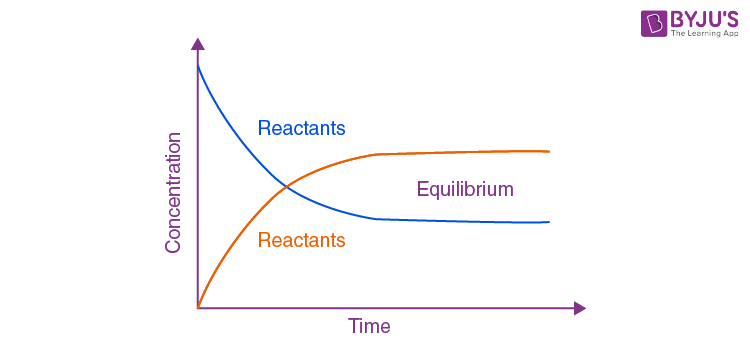State of Chemical Equilibrium

A graph with the concentration on the y-axis and time on the x-axis can be plotted. Once the concentration of both the reactants and the products stops showing change, chemical equilibrium is achieved.

### Why Is Chemical Equilibrium Called Dynamic Equilibrium?

The stage at which the rate of the forward reaction is equal to the rate of the backward reaction is called an equilibrium stage. At this point, the number of reactant molecules converting into products and product molecules into reactants is the same. The same equilibrium can be carried out with the same reactants anywhere with similar conditions with continuous interchanging of molecules, and hence chemical equilibrium is dynamic.

#### Introduction to Chemical Equilibrium#### Chemical Equilibrium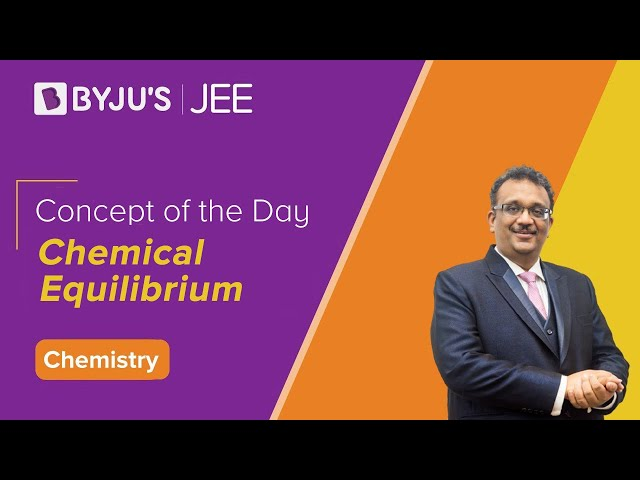## Types of Chemical Equilibrium

There are two types of chemical equilibrium:

• Homogeneous Equilibrium
• Heterogeneous Equilibrium

### Homogenous Chemical Equilibrium

In this type, the reactants and the products of chemical equilibrium are all in the same phase. Homogenous equilibrium can be further divided into two types:

Reactions in which the number of molecules of the products is equal to the number of molecules of the reactants. For example,

• H2 (g) + I2 (g) ⇌ 2HI (g)
• N2 (g) + O2 (g) ⇌ 2NO (g)

Reactions in which the number of molecules of the products is not equal to the total number of reactant molecules. For example,

• 2SO2 (g) + O2 (g) ⇌ 2SO3 (g)
• COCl2 (g) ⇌ CO (g) + Cl2 (g)

### Heterogeneous Chemical Equilibrium

In this type, the reactants and the products of chemical equilibrium are present in different phases. A few examples of heterogeneous equilibrium are given below:

• CO2 (g) + C (s) ⇌ 2CO (g)
• CaCO3 (s) ⇌ CaO (s) + CO2 (g)

Thus, the different types of chemical equilibrium are based on the phase of the reactants and products.

⇒ Check: Ionic Equilibrium

## Factors Affecting Chemical Equilibrium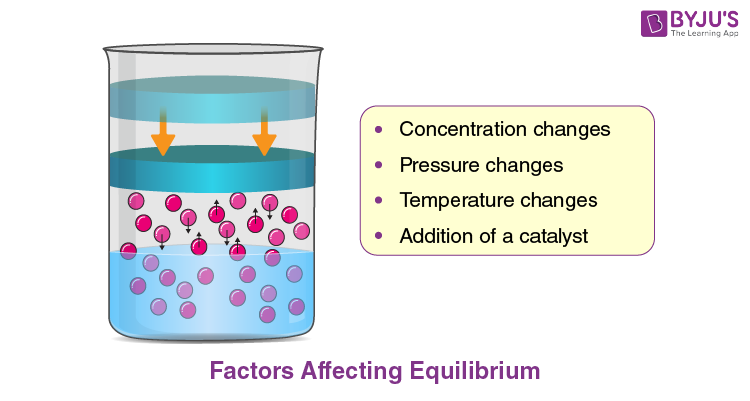Chemical Equilibrium – Factors Affecting Equilibrium

According to Le-Chatelier’s principle, if there is any change in the factors affecting the equilibrium conditions, the system will counteract or reduce the effect of the overall transformation. This principle applies to both chemical and physical equilibrium.

There are several factors like temperature, pressure and concentration of the system that affect equilibrium. Some important factors affecting chemical equilibrium are explained below.

### Change in Concentration

• The concentration of the reactants or products added is relieved by the reaction, which consumes the substance that is added.
• The concentration of reactants or products removed is relieved by the reaction, which is in the direction that replenishes the substance which is removed.
• When the concentration of the reactant or product is changed, there is a change in the composition of the mixture in chemical equilibrium.

#### Effect of Change in Concentration### Change in Pressure

Change in pressure happens due to the change in the volume. If there is a change in pressure, it can affect the gaseous reaction as the total number of gaseous reactants and products are now different. According to Le Chatelier’s principle, in heterogeneous chemical equilibrium, the change of pressure in both liquids and solids can be ignored because the volume is independent of pressure.

#### Effect of Change in Volume of Equilibrium Mixture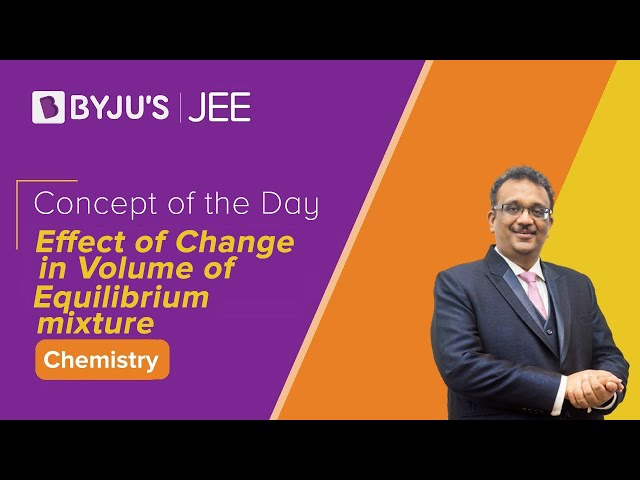### Change in Temperature

The effect of temperature on chemical equilibrium depends upon the sign of ΔH of the reaction and follows Le-Chatelier’s Principle.

• As temperature increases, the equilibrium constant of an exothermic reaction decreases.
• In an endothermic reaction, the equilibrium constant increases with an increase in temperature.

Along with the equilibrium constant, the rate of reaction is also affected by the change in temperature. As per Le Chatelier’s principle, the equilibrium shifts towards the reactant side when the temperature increases in the case of exothermic reactions; for endothermic reactions, the equilibrium shifts towards the product side with an increase in temperature.

### Effect of a Catalyst

A catalyst does not affect the chemical equilibrium, it only speeds up a reaction. In fact, the catalyst equally speeds up the forward as well as the reverse reaction. This results in the reaction reaching its equilibrium faster.

The same amount of reactants and products will be present at equilibrium in a catalysed or non-catalysed reaction. The presence of a catalyst only facilitates the reaction to proceed through a lower-energy transition state of reactants to products.

### Effect of Addition of an Inert Gas

When an inert gas like argon is added to a constant volume, it does not take part in the reaction, so the equilibrium remains undisturbed. If the gas added is a reactant or product involved in the reaction, then the reaction quotient will change.

#### Effect of Addition of Inert Gas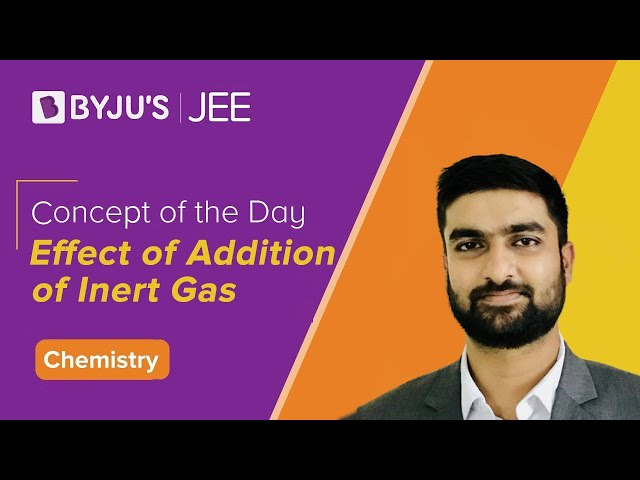## Examples of Chemical Equilibrium

In chemical reactions, reactants are converted into products by the forward reaction, and the products may be converted into reactants by the backward reaction. The two states, reactants and products, are different in composition.

After some time of the start of the reaction, the rate of the forward and the backward reactions may become equal. Then, the number of reactants converted will be formed again by the reverse reaction such that the concentration of reactants and products do not change anymore. Hence, the reactants and products are in chemical equilibrium.

• N2O4 ⇌ 2NO2
• PCl5 ⇌ PCl+PCl2
• N+ H2 ⇌ 2NH3

## Importance of Chemical Equilibrium

It is useful in many industrial processes, like

• Preparation of ammonia by Haber’s process: In this process, nitrogen combines with hydrogen to form ammonia; the yield of ammonia is more at low temperature, high pressure and in the presence of iron as a catalyst.
• Preparation of sulphuric acid by contact process: In this process, the fundamental reaction is the oxidation of sulphur dioxide into sulphur trioxide. This involves chemical equilibrium.

### Equilibrium Solved Questions### Ionic Equilibrium Top 7 Questions## Problems on Chemical Equilibrium

1. The equilibrium constant KP for the reaction N2 (g) + 3H2 (g) ⇌ 2NH3 (g) is 1.6 × 10-4 atm-2 at 400oC. What will be the equilibrium constant of the Chemical equilibrium at 500oC if the heat of the reaction at this temperature range is -25.14 kcal?

Solution:

Equilibrium constants at different temperatures and heat of the reaction are related by the equation,

log KP2 = -25140/2.303 × 2 [773 – 673 / 773 × 673] + log 1.64 × 10-4

log KP2 = -4.835

KP2 = 1.462 × 10-5 atm-2

2. Given the equation, N2 (g) + 3H2 (aq) ⇌ 2NH3 (g). Find Q and determine which direction the reaction will shift in order to reach the state of chemical equilibrium.

Solution:

Given, [N2] = 0.04M, [H2] = 0.09M, and K = 0.040

Since only nitrogen and hydrogen concentration is given, they can be assumed as the reactants and ammonia as the product. Since ammonia concentration is not given, it can be assumed to be zero.

As q is the ratio of the relative concentration of products to reactants, here, Q =0.

Since K = 0.04 is larger than Q, nitrogen and hydrogen will combine to form the product ammonia.

### Chemical Equilibrium – All Concepts and JEE Questions### Equilibrium – Top 15 Most Important and Expected JEE Questions### Chemical Equilibrium One Shot Revision for JEEQ1

### What is the effect of temperature on the equilibrium constant during an exothermic reaction?

With the increase in temperature, the equilibrium constant decreases during an exothermic reaction.
Q2

### What is the effect of a catalyst on a chemical equilibrium?

A catalyst has no effect on the chemical equilibrium.
Q3

### What is the effect of the addition of inert gas during chemical equilibrium?

There is no effect on chemical equilibrium with the addition of inert gas.
Q4

### What is meant by the forward reaction?

A reaction in which the reactants are converted to products is called a forward reaction.
Q5

### What is meant by the backward reaction?

A reaction in which the products are converted back to reactants is called the backward reaction.
Test your knowledge on Chemical Equilibrium